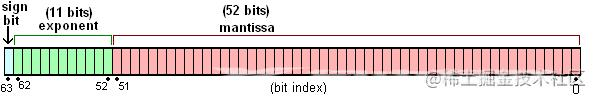﻿ JS中浮点数精度问题的分析与解决方法 - 自由资讯

# JS中浮点数精度问题的分析与解决方法

##### 目录
• 前言
• 问题的发现
• 浮点数运算后的精度问题
• toFixed奇葩问题
• 为什么会产生
• 浮点数的存储
• 浮点数的运算
• 解决方法
• 解决toFixed
• 解决浮点数运算精度
• 附：JS浮点数精度问题的一些实用建议
• 总结

## 前言

最近在做项目的时候，涉及到商品价格的计算，经常会出现计算出现精度问题。刚开始草草了事，直接用toFixed就解决了问题，并没有好好的思考一下这个问题。后来慢慢的，问题越来越多，连toFixed也出现了（允悲），后来经过搜索网上的各种博客和论坛，整理总结了一下。

## 问题的发现

总结了一下，一共有以下两种问题

### 浮点数运算后的精度问题

在计算商品价格加减乘除时，偶尔## 会出现精度问题，一些常见的例子如下：

```// 加法 =====================
0.1 + 0.2 = 0.30000000000000004
0.7 + 0.1 = 0.7999999999999999
0.2 + 0.4 = 0.6000000000000001

// 减法 =====================
1.5 - 1.2 = 0.30000000000000004
0.3 - 0.2 = 0.09999999999999998

// 乘法 =====================
19.9 * 100 = 1989.9999999999998
0.8 * 3 = 2.4000000000000004
35.41 * 100 = 3540.9999999999995

// 除法 =====================
0.3 / 0.1 = 2.9999999999999996
0.69 / 10 = 0.06899999999999999```

### toFixed奇葩问题

在遇到浮点数运算后出现的精度问题时，刚开始我是使用toFixed(2)来解决的，因为在W3school和菜鸟教程（他们均表示这锅不背）上明确写着定义：toFixed()方法可把Number四舍五入为指定小数位数的数字。

但是在chrome下测试结果不太令人满意：

```1.35.toFixed(1) // 1.4 正确
1.335.toFixed(2) // 1.33  错误
1.3335.toFixed(3) // 1.333 错误
1.33335.toFixed(4) // 1.3334 正确
1.333335.toFixed(5)  // 1.33333 错误
1.3333335.toFixed(6) // 1.333333 错误```

使用IETester在IE下面测试的结果却是正确的。

## 为什么会产生

让我们来看一下为什么0.1+0.2会等于0.30000000000000004，而不是0.3。首先，想要知道为什么会产生这样的问题，让我们回到大学里学的复（ku）杂（zao）的计算机组成原理。虽然已经全部还给大学老师了，但是没关系，我们还有百度嘛。

### 浮点数的存储

和其它语言如Java和Python不同，JavaScript中所有数字包括整数和小数都只有一种类型 — Number。它的实现遵循 IEEE 754 标准，使用64位固定长度来表示，也就是标准的 double 双精度浮点数（相关的还有float 32位单精度）。

这样的存储结构优点是可以归一化处理整数和小数，节省存储空间。

64位比特又可分为三个部分：

• 符号位S：第 1 位是正负数符号位（sign），0代表正数，1代表负数

• 指数位E：中间的 11 位存储指数（exponent），用来表示次方数

• 尾数位M：最后的 52 位是尾数（mantissa），超出的部分自动进一舍零## 浮点数的运算

那么JavaScript在计算0.1+0.2时到底发生了什么呢？

首先，十进制的0.1和0.2会被转换成二进制的，但是由于浮点数用二进制表示时是无穷的：

```0.1 -> 0.0001 1001 1001 1001...(1100循环)
0.2 -> 0.0011 0011 0011 0011...(0011循环)```

IEEE 754 标准的 64 位双精度浮点数的小数部分最多支持53位二进制位，所以两者相加之后得到二进制为：

`0.0100110011001100110011001100110011001100110011001100 `

因浮点数小数位的限制而截断的二进制数字，再转换为十进制，就成了0.30000000000000004。所以在进行算术计算时会产生误差。

## 解决方法

针对以上两个问题，网上搜了一波解决方法，基本都大同小异的，分别来看一下。

### 解决toFixed

针对toFixed的兼容性问题，我们可以把toFix重写一下来解决，代码如下：

```// toFixed兼容方法
Number.prototype.toFixed = function(len){
if(len>20 || len<0){
throw new RangeError('toFixed() digits argument must be between 0 and 20');
}
// .123转为0.123
var number = Number(this);
if (isNaN(number) || number >= Math.pow(10, 21)) {
return number.toString();
}
if (typeof (len) == 'undefined' || len == 0) {
return (Math.round(number)).toString();
}
var result = number.toString(),
numberArr = result.split('.');

if(numberArr.length<2){
//整数的情况
}
var intNum = numberArr, //整数部分
deciNum = numberArr,//小数部分
lastNum = deciNum.substr(len, 1);//最后一个数字

if(deciNum.length == len){
//需要截取的长度等于当前长度
return result;
}
if(deciNum.length < len){
//需要截取的长度大于当前长度 1.3.toFixed(2)
}
//需要截取的长度小于当前长度，需要判断最后一位数字
result = intNum + '.' + deciNum.substr(0, len);
if(parseInt(lastNum, 10)>=5){
//最后一位数字大于5，要进位
var times = Math.pow(10, len); //需要放大的倍数
var changedInt = Number(result.replace('.',''));//截取后转为整数
changedInt++;//整数进位
changedInt /= times;//整数转为小数，注：有可能还是整数
}
return result;
//对数字末尾加0
var dotPos = num.indexOf('.');
if(dotPos === -1){
//整数的情况
num += '.';
for(var i = 0;i<len;i++){
num += '0';
}
return num;
} else {
//小数的情况
var need = len - (num.length - dotPos - 1);
for(var j = 0;j<need;j++){
num += '0';
}
return num;
}
}
}```

我们通过判断最后一位是否大于等于5来决定需不需要进位，如果需要进位先把小数乘以倍数变为整数，加1之后，再除以倍数变为小数，这样就不用一位一位的进行判断。

### 解决浮点数运算精度

既然我们发现了浮点数的这个问题，又不能直接让两个浮点数运算，那怎么处理呢？

我们可以把需要计算的数字升级（乘以10的n次幂）成计算机能够精确识别的整数，等计算完成后再进行降级（除以10的n次幂），这是大部分变成语言处理精度问题常用的方法。例如：

```0.1 + 0.2 == 0.3 //false
(0.1*10 + 0.2*10)/10 == 0.3 //true```

但是这样就能完美解决么？细心的读者可能在上面的例子里已经发现了问题：

`35.41 * 100 = 3540.9999999999995`

看来进行数字升级也不是完全的可靠啊（允悲）。

但是魔高一尺道高一丈，这样就能难住我们么，我们可以将浮点数toString后indexOf('.')，记录一下小数位的长度，然后将小数点抹掉，完整的代码如下：

``` /*** method **
*  add / subtract / multiply /divide
* floatObj.multiply(19.9, 100) >> 1990
*
*/
var floatObj = function() {
/*
* 判断obj是否为一个整数
*/
function isInteger(obj) {
return Math.floor(obj) === obj
}

/*
* 将一个浮点数转成整数，返回整数和倍数。如 3.14 >> 314，倍数是 100
* @param floatNum {number} 小数
* @return {object}
*   {times:100, num: 314}
*/
function toInteger(floatNum) {
var ret = {times: 1, num: 0}
if (isInteger(floatNum)) {
ret.num = floatNum
return ret
}
var strfi  = floatNum + ''
var dotPos = strfi.indexOf('.')
var len    = strfi.substr(dotPos+1).length
var times  = Math.pow(10, len)
var intNum = Number(floatNum.toString().replace('.',''))
ret.times  = times
ret.num    = intNum
return ret
}

/*
* 核心方法，实现加减乘除运算，确保不丢失精度
* 思路：把小数放大为整数（乘），进行算术运算，再缩小为小数（除）
*
* @param a {number} 运算数1
* @param b {number} 运算数2
* @param digits {number} 精度，保留的小数点数，比如 2, 即保留为两位小数
*
*/
function operation(a, b, digits, op) {
var o1 = toInteger(a)
var o2 = toInteger(b)
var n1 = o1.num
var n2 = o2.num
var t1 = o1.times
var t2 = o2.times
var max = t1 > t2 ? t1 : t2
var result = null
switch (op) {
if (t1 === t2) { // 两个小数位数相同
result = n1 + n2
} else if (t1 > t2) { // o1 小数位 大于 o2
result = n1 + n2 * (t1 / t2)
} else { // o1 小数位 小于 o2
result = n1 * (t2 / t1) + n2
}
return result / max
case 'subtract':
if (t1 === t2) {
result = n1 - n2
} else if (t1 > t2) {
result = n1 - n2 * (t1 / t2)
} else {
result = n1 * (t2 / t1) - n2
}
return result / max
case 'multiply':
result = (n1 * n2) / (t1 * t2)
return result
case 'divide':
result = (n1 / n2) * (t2 / t1)
return result
}
}

// 加减乘除的四个接口
}
function subtract(a, b, digits) {
return operation(a, b, digits, 'subtract')
}
function multiply(a, b, digits) {
return operation(a, b, digits, 'multiply')
}
function divide(a, b, digits) {
return operation(a, b, digits, 'divide')
}

// exports
return {
subtract: subtract,
multiply: multiply,
divide: divide
}
}();```

如果觉得floatObj调用麻烦，我们可以在Number.prototype上添加对应的运算方法。

## 附：JS浮点数精度问题的一些实用建议

• 考虑到每次浮点数运算的偏差非常小(其实不然)，可以对结果进行指定精度的四舍五入，比如可以parseFloat(result.toFixed(12));
• 将浮点数转为整数运算，再对结果做除法。比如0.1 + 0.2，可以转化为(1*2)/3。
• 把浮点数转化为字符串，模拟实际运算的过程。

综上，建议使用第三种方案，目前已经有了很多较为成熟的库，我们可以根据自己的需求来选择对应的工具。并且，这些库不仅解决了浮点数的运算精度问题，还支持了大数运算，并且修复了原生toFixed结果不准确的问题。

## 总结

到此这篇关于JS中浮点数精度问题的文章就介绍到这了,更多相关JS浮点数精度问题内容请搜索自由互联以前的文章或继续浏览下面的相关文章希望大家以后多多支持自由互联！

上一篇：JavaScript中防抖和节流的实战应用记录
下一篇：没有了
网友评论
相关栏目
﻿